# Real projective three-dimensional space

## Definition

As a topological space, this can be defined in the following equivalent ways:

• The real projective space of dimension 3. It is denoted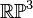$\R\mathbb{P}^3$ or$\mathbb{P}^3\R$. In particular, it is the quotient of the 3-sphere under the antipodal map.
• The underlying topological space of the special orthogonal group$SO(3,\R)$.

Note that real projective spaces are not in general homeomorphic to the underlying spaces of special orthogonal groups.

## Topological space properties

Property Satisfied? Is the property a homotopy-invariant property of topological spaces? Explanation Corollary properties satisfied/dissatisfied
manifold Yes No Based on the definition; in fact, any finite-dimensional real projective space is a manifold. We can also see this from the fact that its double cover, the 3-sphere, is a manifold satisfies: metrizable space, second-countable space, and all the separation axioms down from perfectly normal space and monotonically normal space, including normal, completely regular, regular, Hausdorff, etc.
path-connected space Yes Yes Can be seen directly, or from the fact that its double cover, the 3-sphere, is path-connected. satisfies: connected space, connected manifold, homogeneous space (via connected manifold, see connected manifold implies homogeneous)
simply connected space No Yes It has a double cover, namely the 3-sphere, which is path-connected. In fact, the double cover is simply connected, so the fundamental group of the space is a cyclic group of order two. dissatisfies: weakly contractible space, contractible space
acyclic space No Yes$H_1$ of the space is cyclic of order two (this can be seen from the Hurewicz theorem and the fact that$\pi_1$ is cyclic of order two, or directly using the homology of real projective space). dissatisfies: weakly contractible space, contractible space
rationally acyclic space No Yes The third homology group is isomorphic to$\mathbb{Z}$ dissatisfies: space with Euler characteristic one
space with zero Euler characteristic Yes Yes There are many ways of seeing this:
Euler characteristic of compact connected odd-dimensional manifold is zero, Euler characteristic of compact connected nontrivial Lie group is zero (using the view of it as$SO(3,\R)$), and the fact that its double cover is the 3-sphere (which has Euler characteristic zero) and Euler characteristic of covering space is degree of covering times Euler characteristic of base.
It also follows from the homology of real projective space]]
-
compact space Yes No Its double cover, the 2-sphere, is compact, and compactness is continuous image-closed dissatisfies: compact manifold, compact polyhedron, polyhedron (via compact manifold), compact Hausdorff space, and all properties weaker than compactness

## Algebraic topology

### Homology groups

Further information: homology of real projective space

The homology groups with coefficients in integers are as follows:$H_p(\R\mathbb{P}^3; \mathbb{Z}) = \lbrace \begin{array}{rl} \mathbb{Z}, & \qquad p = 0,3 \\ \mathbb{Z}/2\mathbb{Z}, & \qquad p = 1 \\ 0 & \qquad p = 2 \ \operatorname{or} \ p > 3 \\\end{array}$

The top homology group is$\mathbb{Z}$, indicating that the manifold is orientable, which it is. This is common to all odd-dimensional real projective spaces.

The homology groups with coefficients in a module$M$ over a ring are as follows:$H_p(\R\mathbb{P}^3; M) = \lbrace \begin{array}{rl} M, & \qquad p = 0,3 \\ M/2M, & \qquad p = 1 \\ T & \qquad p = 2 \\ 0 & \qquad p > 3 \\\end{array}$

Here,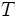$T$ denotes the 2-torsion submodule of$M$. In particular, if$M$ is uniquely 2-divisible (the case for any field of characteristic not equal to 2), we get: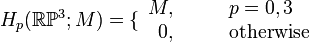$H_p(\R\mathbb{P}^3; M) = \lbrace \begin{array}{rl} M, & \qquad p = 0,3 \\ 0, & \qquad \operatorname{otherwise} \\\end{array}$

### Cohomology groups

Further information: cohomology of real projective space

The cohomology groups with coefficients in integers are as follows: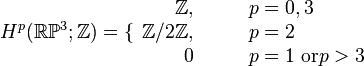$H^p(\R\mathbb{P}^3; \mathbb{Z}) = \lbrace \begin{array}{rl} \mathbb{Z}, & \qquad p = 0,3 \\ \mathbb{Z}/2\mathbb{Z}, & \qquad p = 2 \\ 0 & \qquad p = 1 \ \operatorname{or} p > 3 \\\end{array}$

These can be obtained from the homology groups using the Poincare duality theorem, among other methods.

The cohomology groups with coefficients in a module$M$ over a ring are as follows: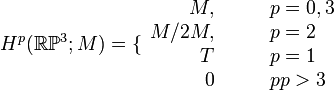$H^p(\R\mathbb{P}^3; M) = \lbrace \begin{array}{rl} M, & \qquad p = 0,3 \\ M/2M, & \qquad p = 2 \\ T & \qquad p = 1 \\ 0 & \qquad p p > 3 \\\end{array}$

Here,$T$ denotes the 2-torsion submodule of$M$. In particular, if$M$ is uniquely 2-divisible (the case for any field of characteristic not equal to 2), we get:$H_p(\R\mathbb{P}^3; M) = \lbrace \begin{array}{rl} M, & \qquad p = 0,3 \\ 0, & \qquad \operatorname{otherwise} \\\end{array}$

### Homotopy groups

Further information: homotopy of real projective space

Value of$k$ General name for homotopy group or set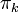$\pi_k$ What is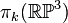$\pi_k(\R\mathbb{P}^3)$?
0 set of path components one-point set, i.e., the space is a path-connected space
1 fundamental group cyclic group:Z2, i.e., the group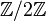$\mathbb{Z}/2\mathbb{Z}$. The universal covering space is the 3-sphere which is simply connected and is a double cover (the quotient map is the identification of antipodal points).
2 second homotopy group trivial group
3 third homotopy group$\mathbb{Z}$ -- group of integers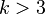$k > 3$ Isomorphic to$\pi_k(S^3)$, i.e., the corresponding homotopy group of the 3-sphere.

### Homology-based invariants

Invariant General description Description of value for real projective space$\R\mathbb{P}^n$ Description of value for$\R\mathbb{P}^2$
Betti numbers The$k^{th}$ Betti number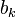$b_k$ is the rank of the torsion-free part of the$k^{th}$ homology group.$b_0 = 1$.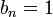$b_n = 1$ if$n$ is odd and$b_n = 0$ if$n$ is even. All other$b_k$s are zero.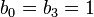$b_0 = b_3 = 1$, all other$b_k$s are zero.
Poincare polynomial Generating polynomial for Betti numbers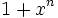$1 + x^n$ if$n$ is odd.$1$ if$n$ is even.$1 + x^3$
Euler characteristic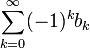$\sum_{k=0}^\infty (-1)^k b_k$$0$ if$n$ is odd.$1$ if$n$ is even. Note that the Euler characteristic is half the Euler characteristic of the sphere$S^n$, which is its double cover. 1